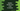# Python program to iterate over the list in reverse orderIn this tutorial, we will learn how to iterate over a list in reverse order. For example, if we have a list [1,2,3,4,5], we will traverse it in the order 5->4->3->2->1. We have different ways to traverse a list in reverse order. This iteration will not change the order of the list and we will not do any modification to the list elements. I will show you different ways to achieve this. Let’s take a look :

The source code for the below examples is available here.

## Iterate over the list in reverse using ‘for’ loop :

loop helps us always while iterating through something. In python, we have range() function to iterate. We can define this method as range([start], stop[, step]).

1. start: It is the starting index of the sequence.
2. stop: The range will run till this index, but it will not include this index.
3. step: Difference between each element of the sequence.

So, in our case, the start will be the index of the last element of the list, stop will be 0 i.e. first element of the list and step will be -1 since we are decrementing the index by 1 on each step.

The program will look like as below :

### Explanation :

The commented numbers in the above program denote the step number below :

1. mylist_ is the list we are using in this program.
2. This for loop will run from index of the last element of the list to the 0th index element of the list. The print statement inside the loop will print out the values. Output:

### Using a while loop :

Similar to a for loop, we can also use while loop to iterate a list in reverse.

### Explanation :

The commented numbers in the above program denote the step number below :

1. We are using the same list as the above example.
2. First, we have calculated the length of the list using the len() method.
3. index variable is initialized with value length of the list - 1. It is used to indicate the current index of the list while iterating.
4. This loop will run until the value of index become 0. Each time, index value is decremented by 1. The print line will print out the current iterating value of the list.

It will print the following output :

## Using [::-1], the simplest way to reverse a list :

We can also reverse a list using only one line like below :

mylist[::-1]_ creates a reversed list and store it in reverselist_ variable. It will print the below output :

### Conclusion :

We have learned three different ways to reverse a list in python. You can use any one of the methods above but the third one is the easiest one. Try the examples above and drop a comment below if you find any problem.